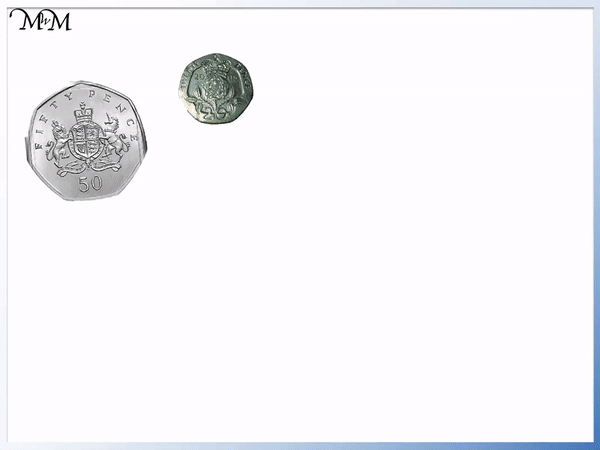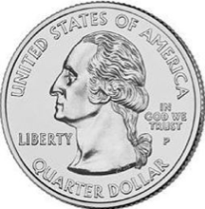# Giving Change

Giving Change• This toy costs 45 pence but we only have a 50p coin.
• We cannot pay 45 pence exactly and so we pay with the 50p coin.
• 50p is 5 pence more than 45p.
• We will be given this 5 pence back as a 5p coin.
• The money we are given back is called change.
• Since we started with 50p and now have 5p, our money has gone down by 45 pence.

Change is the money given back if you pay more than an item costs.

These happens when you do not have the exact amount of money.• We have 10p + 10p + 10p + 5p, which makes 35 pence in total.
• This banana costs 32 pence.
• We have more than 32 pence but we cannot pay for the banana exactly.
• 35 – 32 = 3.
• 35p is 3 pence more than the cost of the banana.
• We are given this 3 pence back as change.
• There is no 3 pence coin and so, the change is given back as a 2p coin plus a 1p coin.# Giving Change with British Money

## What is change?

Money comes in certain values which are written on coins or notes. Because of this it is unlikely that you will always be carrying the exact amount of money needed to pay for something.

If this is the case then we need to pay more than the cost of the item and then get the extra money back.

The money given back is called change. Change is the difference between the cost of the item and the money given to pay for it.

In the example below we have four coins: three 10p coins and one 20p coin.

In total this is 50 pence.

We want to buy a spinning top for 30 pence.We can pay for the spinning top with 30p from our 50 pence. We can pay using the three 10p coins.

In this example, we have 20p left. We were able to pay for it directly with the coins we had and so, we were not given change.

We started with 50 pence and spent 30 pence.

To see how much money we have remaining we subtract the cost of the item from the amount of money we started with.

50 – 30 = 20

We have 20 pence remaining after purchasing the toy.

Here is an example with four coins: three 10p coins and a 5p coin. We have 35p.

We want to buy a banana which costs 32 pence.This time we cannot pay for the banana exactly. If we give three 10p coins, then we spend 30 pence and if we give the remaining 5p coin, we have paid 35 pence.

We give an amount greater than the cost of the banana and so we give 35 pence.

35 is 3 more than 32 and so, we have spent 3 pence too much.

To keep it fair, we are given this 3 pence back as change.

We are given a 1p and 2p coin, worth 3 pence.

In this example of giving change, we have 44 pence.

We want to buy a toy teddy bear which costs 43 pence.We cannot spend exactly 43 pence and so we hand over all of the money.

44 pence is 1 pence more than 43 pence and so, we have spent 1p more than the cost of the bear.

We are given this 1p coin back as change.

Here we have a 50p coin and want to buy a toy car which costs 45 pence.

We can only hand over the 50p coin and wait to receive change.

We can work out the change by thinking ‘How much more than 45 is 50?’.50 is 5 more than 45 and so, we receive 5p back as change.

In this next example we have a 50p coin and a 20p coin. We want to buy an apple which costs 63 pence.

50p plus 20p is a total of 70 pence.

We will need to pay with both coins and wait to receive change.We can think ‘How much more than 63 is 70?’.

70 is 7 more than 63 and so, we receive 7 pence back as change.

7p can be made using a 5 pence coin and a 2 pence coin.Now try our lesson on Counting US Coins: Dimes, Nickels, Pennies & Quarters where we learn about the different US coins.